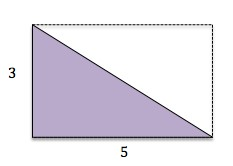### ← Math: Geometry Concepts you don't know will reappear later

You should create an account to save your progress. It only takes a minute!
new term

### area of a triangle

The formula for the area of a triangle is (base × height) / 2. Hint: Remember this is because a triangle can be considered to be half of a rectangle and the area of a rectangle is base × height.

Example: Area of the purple triangle below = (5 × 3)/2 = 7.5.I knew this term I didn't know this term
You have mastered 0 out of 22 concepts
You are reviewing 0 out of 22 concepts
You are learning 0 out of 22 concepts